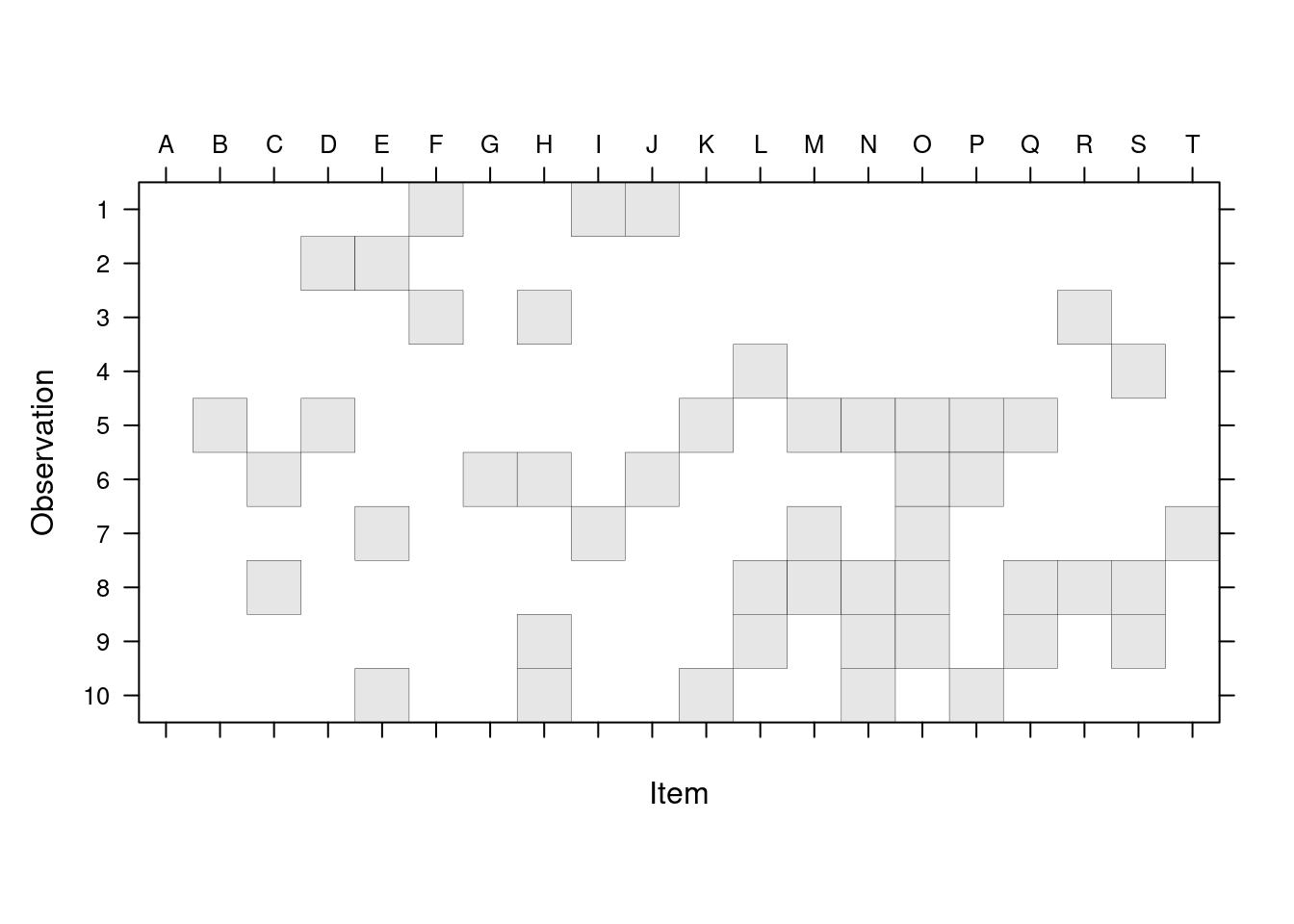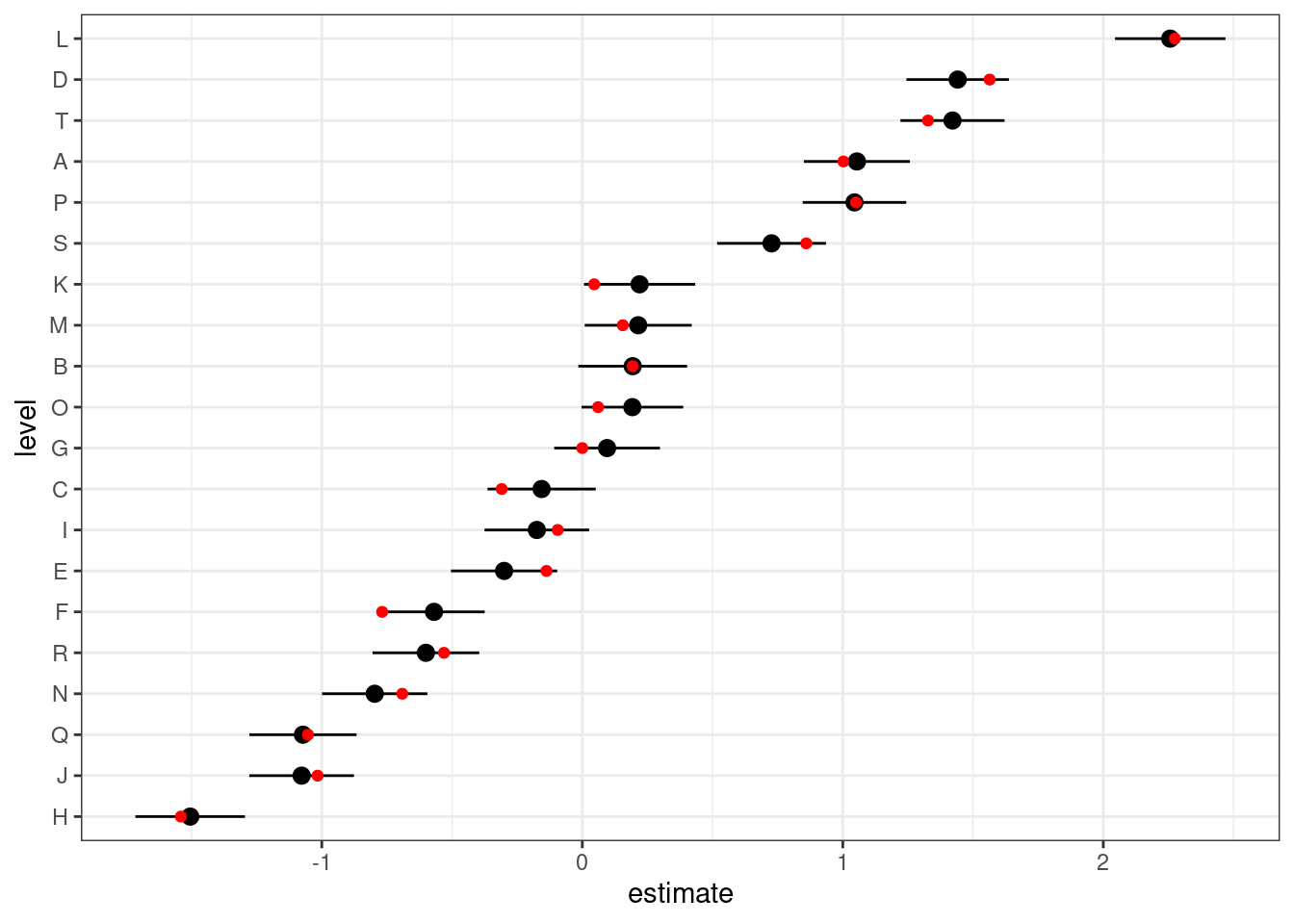Consider a situation where we have multiple items of some sort which have associated effects, but we can’t association observations with single items; instead, each observation is associated with a group of items. (Two examples which have come up are (1) authorship of papers and (2) hockey players on a team.) These are not quite identical to “multi-membership models” (I think), although similar techniques to those shown here could work for multi-membership models.

Load packages (we don’t really need anything beyond lme4; the rest are for convenience/drawing pictures).

library(lme4)
library(broom.mixed)
library(ggplot2); theme_set(theme_bw())
library(Matrix)
library(dplyr); library(tidyr)

Construct a simulated example: first, simulate the design (structure).

nm <- 20
nobs0 <- 500
set.seed(101)
## choose items for observations
W1 <- matrix(rbinom(nobs0*nm, prob=0.25, size=1),nrow=nobs0,ncol=nm)
dimnames(W1) <- list(NULL,LETTERS[seq(nm)])
W1[1:5,]
##      A B C D E F G H I J K L M N O P Q R S T
## [1,] 0 0 0 0 0 1 0 0 1 1 0 0 0 0 0 0 0 0 0 0
## [2,] 0 0 0 1 1 0 0 0 0 0 0 0 0 0 0 0 0 0 0 0
## [3,] 0 0 0 0 0 1 0 1 0 0 0 0 0 0 0 0 0 1 0 0
## [4,] 0 0 0 0 0 0 0 0 0 0 0 1 0 0 0 0 0 0 1 0
## [5,] 0 1 0 1 0 0 0 0 0 0 1 0 1 1 1 1 1 0 0 0
table(rowSums(W1))
##
##   1   2   3   4   5   6   7   8   9  10  11
##  11  40  63  85 106  85  52  37  11   7   3

The first 10 observations:

image(Matrix(W1),ylim=c(1,10),sub="",ylab="Observation",
xlab="Item",
## draw tick labels at top
scales=list(at=1:20,x=list(labels=colnames(W1),
alternating=2)))Since we chose which items/individuals to include for each observation randomly and independently (Bernoulli with probability 0.25), we have a highly variable number of individuals present for different observations (1-11). This would be realistic for some examples (authorship), unrealistic for others (hockey) … I don’t think it really makes much difference computationally (statistically, having some observations with a single member must make estimation more powerful …) The 0/1 matrix (indicator variable for whether item $$i$$ is included in observation $$j$$) is convenient, and will turn out to be the form we need for inclusion in the model. It should be fairly straightforward to convert other forms (e.g. a list of sets of items associated with each observation) to this form …

Now simulate the response variable.

b <- rnorm(nm)  ## item-level effects
beta <- c(1,2)
M <- data.frame(x=runif(nobs0))
X <- model.matrix(~x, data=M)
## n.b. get in trouble if we don't add residual error
## (theta is scaled relative to residual error)
## here, use theta=sigma=1
M$y <- c(X %*% beta) + c(W1 %*% b) +rnorm(nobs0,sd=1) ## we'll need this later M$fake <- rep(LETTERS[seq(nm)],length.out=nobs0)

## modular/from scratch in lme4

Fit (see ?modular):

## helpful to specify a factor with the right levels:
## actual values are unimportant since we will specify Zt/Ztlist directly
lmod <- lFormula(y~x+(1|fake), data=M)
lmod$reTrms$Zt <- lmod$reTrms$Ztlist[] <- Matrix(t(W1))
devfun <- do.call(mkLmerDevfun, lmod)
opt <- optimizeLmer(devfun)
m1 <- mkMerMod(environment(devfun), opt, lmod$reTrms, fr = lmod$fr)

Results look OK (correct fixed effects, item and residual variance estimated):

m1
## Linear mixed model fit by REML ['lmerMod']
## REML criterion at convergence: 1506.767
## Random effects:
##  Groups   Name        Std.Dev.
##  fake     (Intercept) 0.9580
##  Residual             0.9944
## Number of obs: 500, groups:  fake, 20
## Fixed Effects:
## (Intercept)            x
##      0.8534       2.2106

The conditional modes by item look good:

dd <- tidy(m1, effects="ran_vals")
dd <- transform(dd, level=reorder(level,estimate))
truth <- data.frame(level=LETTERS[seq(nm)],estimate=b)
ggplot(dd,aes(x=level,y=estimate))+
geom_pointrange(aes(ymin=estimate-2*std.error,
ymax=estimate+2*std.error))+coord_flip()+
geom_point(data=truth,colour="red")sos::findFn("{multiple membership}") ## GPvam, R2MLwiN, RealVAMS, ...
• r-sig-mixed-models posts (here, here)
• mm(), mmc() in the brms package
• mult.memb() in the MCMCglmm() package

## function encapsulation

Suppose we have a real data frame M and a (sparse) weight matrix W (or? a list L of weight vectors?

##' @param formula mixed model formula
##' @param data data frame (possibly but not necessarily containing factors
##' @param memb_mat list of weights matrices  with which to replace Zt components
lmer_multimemb <- function(formula,data,memb_mat=list(),...) {
## FIXME: pass ... through appropriately
## FIXME: test dimensions
mnms <- names(memb_mat)
fb <- findbars(formula)
gvars <- vapply(fb, function(x) deparse(x[]), character(1))
Ztlist <- list()
for (i in seq_along(fb)) {
fbnm <- deparse(fb[[i]])
## find corresponding random-effects term
w <- which(mnms==gvars[i])
if (length(w)>0) {
M <- Matrix::Matrix(memb_mat[[w]])
## extract LHS (effect)
form <- as.formula(substitute(~z,list(z=fb[[i]][])))
## construct model matrix & compute Khatri-Rao product
X <- model.matrix(form,data=data)
Zt <- Matrix::KhatriRao(t(M), t(X), make.dimnames=TRUE)
## FIXME: mess with names?
Ztlist[[fbnm]] <- Zt
## if necessary, add factor to data
if (!gvars[[i]] %in% names(data)) {
## if the factor has non-trivial ordering, it should be included
## in the data.  Do we have to worry about ordering of Z? test!
data[[gvars[i]]] <- factor(colnames(memb_mat[[w]]))
}
} ## if  (length(w)>0)
} ## for i in seq(fb)
## call lFormula  (FIXME: allow glFormula)
lmod <- lFormula(formula,data=data)
## substitute new Ztlist elements
for (m in names(Ztlist)) {
lmod$reTrms$Ztlist[[m]] <- Ztlist[[m]]
}
lmod$reTrms$Zt <- do.call(rbind,lmod$reTrms$Ztlist)
## finish fitting
devfun <- do.call(mkLmerDevfun, lmod)
opt <- optimizeLmer(devfun)
m1 <- mkMerMod(environment(devfun), opt, lmod$reTrms, fr = lmod$fr)
return(m1)
}

This seems to work …

(m2 <- lmer_multimemb(formula=y ~ x + (1|team),
data=M,
memb_mat=list(team=W1)))
## Linear mixed model fit by REML ['lmerMod']
## REML criterion at convergence: 1506.767
## Random effects:
##  Groups   Name        Std.Dev.
##  team     (Intercept) 0.9580
##  Residual             0.9944
## Number of obs: 500, groups:  team, 20
## Fixed Effects:
## (Intercept)            x
##      0.8534       2.2106

## Data formats

We want to allow users to conveniently transform whatever data formats they have to a sparse incidence matrix.

### memberships in multiple columns

Maybe it’s in multiple columns (as in this example from Harold Doran):

tmp <- data.frame(id = 1:5,
tch1 = c(10,10,11,11,12),
tch2 = c(77,15,NA,10,11),
tch3 = c(NA, NA, 77, 12, 10),
y = rnorm(5), x = rnorm(5))
## list of teachers
all_tch <- with(tmp,sort(unique(na.omit(c(tch1,tch2,tch3)))))
## empty (sparse) matrix with dimnames
W2 <- Matrix(0,nrow=nrow(tmp),ncol=length(all_tch),
dimnames=list(as.character(tmp$id),as.character(all_tch))) ## melt all teacher columns to long/combined format all_prs0 <- na.omit(reshape2::melt(tmp[,1:4],id="id")) all_prs1 <- all_prs0[,c("id","value")] ## convert to numeric indices all_prs2 <- all_prs1 all_prs2[] <- lapply(all_prs2,function(x) as.numeric(factor(x))) ## convert to two-column matrix all_prs2 <- as.matrix(all_prs2) W2[all_prs2] <- 1 ## weights ## row_ID, col_ID, weight as three columns As pointed out by Robyn Wimmers, reshape2::dcast is handy if your data are in a three-column format (row_label, col_label, weight). It’s marginally less efficient because it initially generates a dense matrix. ## convert from the format we have above all_prs3 <- all_prs1 all_prs3[] <- lapply(all_prs3, factor) all_prs3$wts <- 1
## now data frame -> matrix
W3 <- reshape2::dcast(all_prs3,id~value, value.var="wts", fill=0)
idvars <- W3$id W <- Matrix(as.matrix(W3[,-1])) ## drop 'id' column and sparsify rownames(W3) <- as.character(idvars) # glmmTMB Can we do this with glmmTMB? (need modular branch for now: remotes::install_github("glmmTMB/glmmTMB/glmmTMB@modular")) library(glmmTMB) gt0 <- glmmTMB(y~x+(1|fake), data=M, doFit = FALSE) gt1 <- fitTMB(gt0, doOptim = FALSE) ## now hack data gt1$env$data$Z <- as(Matrix(W1), "TsparseMatrix")
## rebuild TMB object
gt1 <- with(gt1\$env,
parameters,
map = map,
random = random,
silent = silent,
DLL = "glmmTMB"))
fit <- with(gt1, nlminb(par, objective = fn, gr = gr))
m3 <- finalizeTMB(gt0, gt1, fit)
L1 <- (list(raw_lme4 = m1, multimemb = m2, glmmTMB = m3)
|> purrr::map_dfr(tidy, effects = "fixed", .id = "model")
|> select(model, term, estimate, std.error)
)
(L1 |> select(-std.error)
|> pivot_wider(id_cols = "term", names_from = "model",
values_from = "estimate")
)
## # A tibble: 2 × 4
##   term        raw_lme4 multimemb glmmTMB
##   <chr>          <dbl>     <dbl>   <dbl>
## 1 (Intercept)    0.853     0.853   0.853
## 2 x              2.21      2.21    2.21
(L1 |> select(-estimate)
|> pivot_wider(id_cols = "term", names_from = "model",
values_from = "std.error")
)
## # A tibble: 2 × 4
##   term        raw_lme4 multimemb glmmTMB
##   <chr>          <dbl>     <dbl>   <dbl>
## 1 (Intercept)    0.146     0.146   0.145
## 2 x              0.158     0.158   0.158

## from package

JP van Paridon and Phillip Alday have a package on GitHub that provides methods to fit these kinds of problems. Don’t quite know how to adapt to this example at the moment …

## NOT WORKING YET
## slightly naughty
while (!require("lmerMultiMember")) {
remotes::install_github("https://github.com/jvparidon/lmerMultiMember")
}
m0 <- lmerMultiMember::lmer(y~x+(1|fake), memberships = list(fake = W1), data=M)
## subscript too long ..

Session info:

sessionInfo()
## R Under development (unstable) (2023-04-07 r84197)
## Platform: x86_64-pc-linux-gnu (64-bit)
## Running under: Pop!_OS 22.04 LTS
##
## Matrix products: default
## BLAS:   /usr/local/lib/R/lib/libRblas.so
## LAPACK: /usr/lib/x86_64-linux-gnu/openblas-pthread/liblapack.so.3;  LAPACK version 3.10.0
##
## locale:
##   LC_CTYPE=en_CA.UTF-8       LC_NUMERIC=C
##   LC_TIME=en_CA.UTF-8        LC_COLLATE=en_CA.UTF-8
##   LC_MONETARY=en_CA.UTF-8    LC_MESSAGES=en_CA.UTF-8
##   LC_PAPER=en_CA.UTF-8       LC_NAME=C
##  LC_MEASUREMENT=en_CA.UTF-8 LC_IDENTIFICATION=C
##
## time zone: America/Toronto
## tzcode source: system (glibc)
##
## attached base packages:
##  stats     graphics  grDevices datasets  utils     methods   base
##
## other attached packages:
##  glmmTMB_1.1.7.9000     tidyr_1.3.0            dplyr_1.1.1
##  ggplot2_3.4.1          broom.mixed_0.2.9.4    lme4_1.1-32
##  Matrix_1.5-4           lmerMultiMember_0.11.4
##
## loaded via a namespace (and not attached):
##   tidyselect_1.2.0    farver_2.1.1        fastmap_1.1.1
##   TH.data_1.1-1       digest_0.6.31       estimability_1.4.1
##   lifecycle_1.0.3     survival_3.5-5      processx_3.8.0
##  magrittr_2.0.3      compiler_4.4.0      rlang_1.1.0
##  sass_0.4.5          tools_4.4.0         utf8_1.2.3
##  yaml_2.3.7          knitr_1.42          prettyunits_1.1.1
##  labeling_0.4.2      pkgbuild_1.4.0      curl_5.0.0
##  plyr_1.8.8          multcomp_1.4-23     withr_2.5.0
##  purrr_1.0.1         numDeriv_2016.8-1.1 desc_1.4.2
##  grid_4.4.0          fansi_1.0.4         xtable_1.8-4
##  colorspace_2.1-0    future_1.32.0       globals_0.16.2
##  emmeans_1.8.5       scales_1.2.1        MASS_7.3-58.3
##  cli_3.6.1           mvtnorm_1.1-3       rmarkdown_2.21
##  crayon_1.5.2        generics_0.1.3      remotes_2.4.2
##  reshape2_1.4.4      minqa_1.2.5         cachem_1.0.7
##  stringr_1.5.0       splines_4.4.0       parallel_4.4.0
##  vctrs_0.6.1         sandwich_3.0-2      boot_1.3-28.1
##  jsonlite_1.8.4      callr_3.7.3         listenv_0.9.0
##  jquerylib_0.1.4     glue_1.6.2          parallelly_1.35.0
##  nloptr_2.0.3        codetools_0.2-19    ps_1.7.4
##  stringi_1.7.12      gtable_0.3.3        munsell_0.5.0
##  tibble_3.2.1        bspm_0.3.10         furrr_0.3.1
##  pillar_1.9.0        htmltools_0.5.5     R6_2.5.1
##  TMB_1.9.1           rprojroot_2.0.3     evaluate_0.18
##  lattice_0.21-8      highr_0.10          backports_1.4.1
##  broom_1.0.4         bslib_0.4.2         Rcpp_1.0.10
##  coda_0.19-4         nlme_3.1-160.9000   xfun_0.38
##  zoo_1.8-11          forcats_1.0.0       pkgconfig_2.0.3